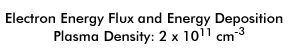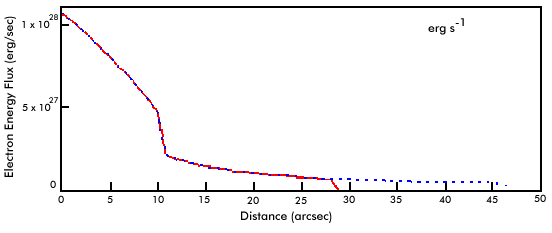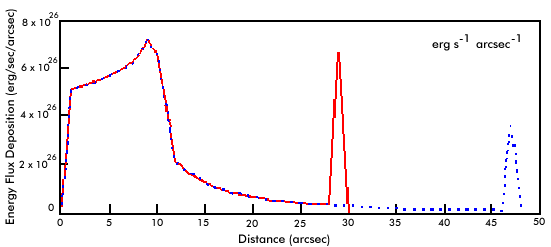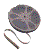# Where is the Energy Deposited?

The energy lost by the injected electrons goes into heating the thermal plasma in the loop. Where in the loop is this energy deposited? How much energy is deposited there? In our model we assumed that the plasma in the loop has a constant temperature and density. Significant energy deposition could change this, however. Also, any increase in the plasma temperature or density will enhance the thermal bremsstrahlung from the loop.

The figures in the table below show the electron energy flux and the energy deposition per unit length as a function of distance (in arc seconds) from the top of the cusp. The solid red curve is for the left leg of the loop and the dashed blue curve is for the right leg. For this plasma density the energy deposited into the cusp region (distance less than 10.7 arcsec) is seen to exceed the energy deposited into the footpoints.Is the energy deposited into the loop plasma significant? We can easily calculate the thermal energy content of our 20 million degree plasma with the adopted density of 2 x 1011 cm-3. The energy contained in a slab of the loop plasma one arc second thick is on the order of 3 x 1027 ergs. The bottom figure shows that the energy deposited into each arc second length of the cusp is as high as 7 x 1026 ergs per second. Therefore, in only a few seconds the energy deposited into the cusp by the injected electrons is comparable to the initial energy content. The actual change in the temperature and density of the cusp plasma depends upon the balance between the collisional heating and energy losses by the plasma. This simple calculation shows that the heating is likely to be significant, however.

The electron energy deposited into the footpoints of the loop primarily heats the cooler, denser plasma in the chromosphere. This heated plasma will most likely expand up into the loop, changing the properties of the loop plasma above the footpoints as well. This expansion of chromospheric plasma up into the corona during a flare is generally called chromospheric evaporation.View a video animation showing how the electron energy flux and energy deposition per unit length change as the plasma density in the loop increases from 1 x 109 cm-3 to 5 x 1012 cm-3. The video is available in AVI (1000 K) and MPEG (348 K) formats.